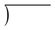top of page# What's next?

Proper and Improper Fractions

# Proper Fractions

Bottom (denominator) is greater than the top (numerator) number. So its value will be below one.

Examples:

1/4 = 0.25

5/16 = 0.3125

11/32 = 0.34375

3/8 = 0.375

7/16 = 0.4375

1/2 = 0.50

9/16 = 0.5625

5/8 = 0.625

11/16 = 0.6875

3/4

13/16

7/8

15/16

# Improper Fractions

Bottom (denominator) is greater than the top (numerator) number. So its value will be greater than one.

Examples: 1/4, 1/10, 4/5, 9/10, 234/300# Food for thought.

### Time24 hours in 1 day.

60 minutes in 1 hour.

60 seconds in 1 minute.

3600 seconds = 1 hour.

86400 seconds = 1 day.

6 hours is 6/24 of a day.

6/24 is same as 0.25.

0.25 = 1/4.

1/4 = 25%

Do you sleep more than 25% of the day away?

1    x 0  = 0

1  x 1 = 1

1  x 6 = 6

2 x 3 = 6

# There are also a few ways to write this.

12           6      12

÷ 6      ÷ 12    ÷ 2

2       0.5        65

3   15

These structures can also be described like this:

15 ÷ 3  = 5

Dividend ÷ Divisor = Quotient

15 / 3 = 5

Dividend / Divisor = Quotient

15    Dividend

÷ 3     Divisor

5     Quotient5

3   15Quotient

Divisor    Dividend